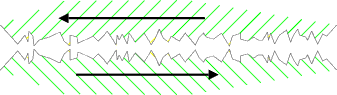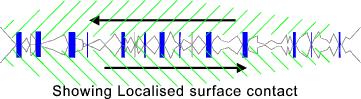Tribology_Index
Coefficients of Friction

Surface Friction

Introduction

All contacting surfaces are directly affected by surface friction.  Surface friction allows control of all forms of motion.  The notes below provide some background into the theory on the factors affecting the coefficient of surface friction.  In practice the coefficient of friction is generally derived from test rigs.  The actual coefficient of friction results as much from the surface conditions as from the materials involved

Classical Friction

The notes below relate to a classical basis for surface friction attributed to Coulomb

At the microscopic level the surface of any solid, no matter how polished, is like the landscape of Switzerland.  Two mating surfaces are in contact only on the tips of the asperities..When two metal surfaces are brought together the area of contact area is actually extremely small so the contact pressure is very high.    Even at surface loads some plastic deformation occurs at the contact points, while the general surface metal is hardly affected.   As the normal load is increased the contact points deform and fracture, thus increasing the real area of contact.   The resulting real area of contact is much less than the apparent area which remains unchanged.Assuming that the plastic yield stress is constant ( Sy ), the real area of contact for each point taking a proportion of the load A i = Fi / S y, so the total real contact area is:

The total contact

A t = F 1 / S y + F 2 / S y.....F i / S y = F t /S y

The real area of contact is dependent on the load and is independent of the area of the surfaces..

To cause relative motion requires the shearing of the contact areas.   The shear force required F s = A t. S s where S s= the shear strength of the material
Therefore the coefficient of friction m = Fs / Ft =( A t. S s ) / ( A t. S y )

m = S s / S y

Considering different failure regimes for a surface to fail in shear the associated direct stress is as follows

 Von Mises (Failure theories )... S s = S y / Ö 3 That is m = 0,58 Tresca (Failure theories ).... S s = S y / 2 .. That is m = 0,5 Actual experience for steel (Shear Stress vs Tensile Stress ) Shear strength = 0.75 Tensile Strength ..That is m = 0,75

A variation on this theory is that the area of contact is Ft / H (hardness) which is about 3 time the plastic yield value s y. This reduces the values for the coefficient of friction by a factor of 3. The resulting value for coefficient of friction based on this variation of the theory is about 0,2 for all metals..

The above notes relate to a very simplified theory on surface friction. In reality most metal surfaces are covered by am oxide layer and a thin film, water vapour and other absorbed impurities.   The localised point contact metal surfaces weld together to form joints.   The shear strength of these microscopic joints is heavily dependent on the shear strength of the surface films.   It is the surface layers that determines the coefficient of friction and not, in general, the parent metal.

Other effects also have an effect on the surface friction values including

 Surface adhesion between pure metal surfaces which probably contributes little to surface friction under normal conditions because of the effect of surface films and contaminants. In vacuum conditions surface adhesion probably has a significant effect on the friction. Ploughing- This is the ploughing of one surface by the asperities on the other surface Elastic deformation of the surfaces as relative movement takes place Junction growth resulting in an increase in the contact area as relative motion takes place

If the surface films can be eliminated then the coefficient of friction can be increased by an order of magnitude. This has be done experimentally by heating test metals in a vacuum

 Links to Friction Information Fund'ls of Friction and Wear of Automobile Brake Materials. ...Paper Download ..Very informative document Introduction to Tribology -Friction ...Extensive and very clear relevant notes Classical Friction ...A very simple and clear description of what determines the coefficient of friction. Wikipedia Friction Notes ...Comprehensive and informative notes.

Tribology_Index
Coefficients of Friction